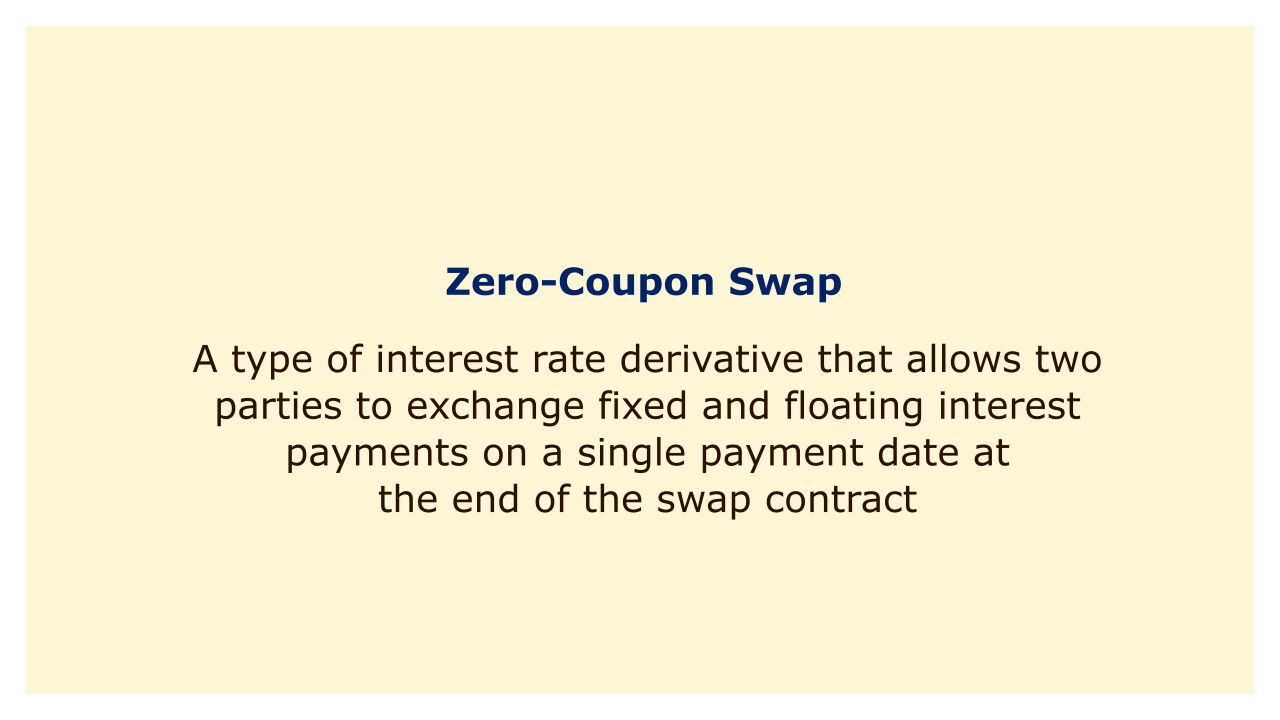# Zero-Coupon SwapImage: Moneybestpal.com

### A zero coupon swap (ZCS) is a type of interest rate derivative that allows two parties to exchange fixed and floating interest payments on a single payment date at the end of the swap contract. Unlike a regular interest rate swap, which involves periodic payments throughout the life of the swap, a ZCS only has one payment date for each leg.

A ZCS can be useful for investors who want to hedge their exposure to interest rate fluctuations or to take advantage of different market conditions. For example, an investor who expects interest rates to fall may enter into a ZCS to lock in a fixed rate of return, while an investor who expects interest rates to rise may enter into a ZCS to benefit from a floating rate of return.

#### How Does a Zero Coupon Swap Work?

A ZCS consists of two legs: a fixed leg and a floating leg. The fixed leg pays a predetermined amount of interest on a notional principal at the end of the swap contract. The floating leg pays an amount of interest that is based on a reference rate, such as LIBOR, on the same notional principal at the end of the swap contract.

The interest payments on both legs are calculated using compound interest, which means that the interest earned or paid in each period is added to the principal for the next period. This makes the calculation of the final payment more complicated than a regular interest rate swap, which uses simple interest.

The value of a ZCS depends on the difference between the fixed and floating interest rates, as well as the time to maturity and the discount factors. A ZCS can be valued by finding the present value of each leg and then subtracting them. The present value of each leg can be found by discounting the future payment by an appropriate discount factor.

#### Example of a Zero Coupon Swap

Suppose that two parties, A and B, enter into a ZCS with a notional principal of \$100 million and a maturity of five years. Party A agrees to pay party B a fixed rate of 5% compounded annually, while party B agrees to pay party A a floating rate of LIBOR compounded annually. Assume that LIBOR is 4% at the start of the swap and 6% at the end of the swap.

The fixed leg payment for party A is:

\$100 million x (1 + 0.05)^5 = \$127.63 million

The floating leg payment for party B is:

\$100 million x (1 + 0.04) x (1 + 0.06)^4 = \$126.53 million

The net payment for party A is:

\$127.63 million - \$126.53 million = \$1.1 million

Party A pays party B \$1.1 million at the end of the swap contract.

In this example, party A benefits from entering into the ZCS because it pays less than it would have paid if it had borrowed at a floating rate. Party B loses from entering into the ZCS because it receives less than it would have received if it had lent at a fixed rate.
Tags